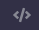# Could anyone help me with this problem?

My C++ project:

``````#include <iostream>
#include <cmath>
using namespace std;

int main(){
int c, TheSum, TheProduct;
int a = 250;
int b = 250;
while (a <= 499){
for(int a; b <= 499; a++){
float c = sqrt(pow(a,2)+pow(b, 2));
TheSum = a+b+c;
if (TheSum == 1000){
TheProduct = a*b*c;
cout << TheProduct;
}
}
b++;
}
cout << TheProduct;
return 0;

}
``````

Could you tell me how much time will this program take to finish?
Thanks

(Edited for readability. You can use this button to preserve your formatting:)